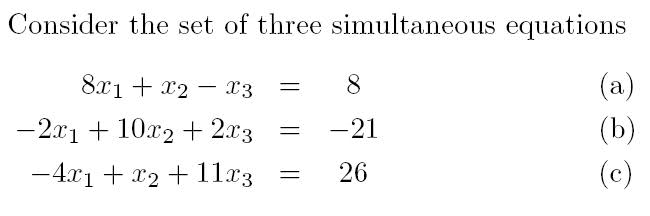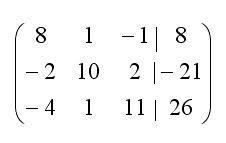# Guassian Elimination (Row reduction) of 3 simultaneous eqn.

## Homework Statement

I have been given the 3 following simultaneous equationsand the question is:
"(a) Solve the equations using Gaussian elimination, showing all your working."

fairly simple topic i know, but i cant get the answer :/

## The Attempt at a Solution

Had a few attempts at it.. heres one of them:

Heres my augmented matrix:I switched Row 1 (R1) with Row 2 (R2)any from there i can work out x1, x2, x3 etc... but i keep getting reidiculous numbers. And when i check them in the 1st set of equations given, they obviously come out to be wrong.

I.e for this attempt i get

x3 = 517/210
x2 = -2297/1230
x1 = -19.408

What am I doing wrong? Any help is much appreciated
Thanks
50